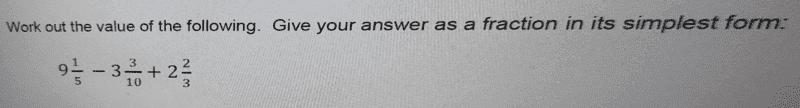Gold Member
Homework Statement:
Kindly see the attachment below:
Relevant Equations:now a bit confusing here, i always use Bodmas in that case,
##9\frac {1}{5}##-[##3\frac {3}{10}##+##2\frac {2}{3}]##... is this correct and what if i re arrange to
##9\frac {1}{5}##+##2\frac {2}{3}##-##3\frac {3}{10}##...
input guys...cheers

i have seen my mistake  is wrong...

Last edited:

Homework Helper
Gold Member
2022 Award
As far as I'm concerned the expression is ambiguous. I'm not convinced there is a universal standard for pluses and minuses.

Gold Member
perok hello, meaning what...i would like to get your opinion.
the negative sign applies to ##3\frac{3}{10}##, that's how i learned ...

Homework Helper
Gold Member
2022 Award
Meaning I don't consider it a well-defined mathematical expression.

Homework Helper
Gold Member
The way I know it is (9+1/5)-(3+3/10)+(2+2/3).

•hmmm27 and SammyS
Gold Member
I don't see how this could be confusing or ambiguous. Just imagine a practical problem.

You have ##9\frac{1}{5}## liters of water in a bucket. Remove ##3\frac{3}{10}## liters from the bucket and add ##2\frac{2}{3}## liters. What is the new volume of water in the bucket?

Say I slightly change the problem. You have ##9\frac{1}{5}## liters of water in a bucket. Add ##3\frac{3}{10}## liters to the bucket and remove ##2\frac{2}{3}## liters. What is the new volume of water in the bucket? Do you apply the negative sign to the fraction part? Of course you do. It wouldn't make sense otherwise. If you remove the whole part, you also remove the fraction part.

This type of fraction notation is defined as a mixed numeral.

Last edited:
•chwala, DaveE, jim mcnamara and 4 others
Gordianus
When I was a kid in elementary school (more than 50 years ago), teachers used to say "fractions (rational number) should only be used to report numbers smaller than one". Thus, if we had 7/5, we were forced to write ##1\frac{2}{5}##. This "saved" the rule we have been given. Casio calculators include this feature and keep alive this old stuff.

Gold Member
When I was a kid in elementary school (more than 50 years ago), teachers used to say "fractions (rational number) should only be used to report numbers smaller than one". Thus, if we had 7/5, we were forced to write ##1\frac{2}{5}##. This "saved" the rule we have been given. Casio calculators include this feature and keep alive this old stuff.
Why would they say that? They're both valid notations, aren't they?

•SammyS
Gordianus
This happened in elementary school. Teachers weren't aware 7/5 is a valid rational number. Moreover, the idea of a "rational number" wasn't popular at that level. We only had "fractions" that, as I already said, were reserved for numbers smaller than one. But this is history.

•chwala and DaveE
Mentor
The way I know it is (9+1/5)-(3+3/10)+(2+2/3).
Yes. This is an expression that involves what are called mixed fractions. Your interpretation is exactly how I see the problem as well.
This happened in elementary school. Teachers weren't aware 7/5 is a valid rational number.
Elementary school teachers who teach mathematics, or even plain old arithmetic, aren't always much up to speed in mathematics. 7/5 is an example of an improper fraction. It certainly is a rational number, and there's no definition that I'm aware of that requires rational numbers to have numerators that are less than their denominators.

•jim mcnamara, Delta2 and chwala
Keith_McClary
I can only refer you to Wikipedia:•chwala
Homework Helper
Gold Member
Homework Statement:: Kindly see the attachment below:

View attachment 284247

now a bit confusing here, i always use Bodmas in that case,
##9\frac {1}{5}##-[##3\frac {3}{10}##+##2\frac {2}{3}]## is this correct and what if i re arrange to
##9\frac {1}{5}##+##2\frac {2}{3}##-##3\frac {3}{10}##
input guys...cheers

i have seen my mistake the first fraction is wrong...
To say what has already been said slightly differently. BODMAS or no BODMAS it is always true that ##9\frac{1}{5} =\frac{46}{5}##, ##3\frac{3}{10}=\frac{33}{10}## and ##2\frac{2}{3}=\frac{8}{3}##.

It follows that ##9\frac{1}{5}-3\frac{3}{10}+2\frac{2}{3}=\frac{46}{5}-\frac{33}{10}+\frac{8}{3}##.

•chwala
Homework Helper
Gold Member
The way I know it is (9+1/5)-(3+3/10)+(2+2/3).
Yes This is the one. Nothing about the expression is ambiguous.

Last edited by a moderator:
•Delta2
Homework Helper
Gold Member
I can only refer you to Wikipedia:Basic Pre-Algebra stuff

Something that can be done is like this:
a-b+c
a+(-b)+c
Sometimes this helps but is often not necessary.

•chwala
Homework Statement:: Kindly see the attachment below:

View attachment 284247

now a bit confusing here, i always use Bodmas in that case,
##9\frac {1}{5}##-[##3\frac {3}{10}##+##2\frac {2}{3}]##... is this correct and what if i re arrange to
##9\frac {1}{5}##+##2\frac {2}{3}##-##3\frac {3}{10}##...
input guys...cheers

i have seen my mistake  is wrong...

9_1/5 - 3_3/10+ 2_2/3

Convert each mixed fraction to an improper fraction.

9_1/5 = 46/5

3_3/10= 33/10

2_2/3 = 8/3

We now have (46/5) - (33/10) + (8/3).

LDC = 10

Take it from here.

•chwala
Homework Helper
Gold Member
Some variations are possible as long as no property is violated.

(9+1/5)-(3+3/10)+(2+2/3)
or
9&1/5-3&3/10+2&2/3

Since they are all put together by "addition", you can arrange as
9-3+2 +1/5 - 3/10 + 2/3

Dealing with the whole numbers separately,
8 + 1/5 -3/10 + 2/3

Using some number familiarity, see that common denominator there should be 30...
8 + 6/30 - 9/30 +20/30

8+17/30

•chwala and nycmathguy
In my reply which cannot be edited now, the LCD should be 30 not 10. It is a typo.

9_1/5 - 3_3/10+ 2_2/3

Convert each mixed fraction to an improper fraction.

9_1/5 = 46/5

3_3/10= 33/10

2_2/3 = 8/3

We now have (46/5) - (33/10) + (8/3).

LDC = 30

Take it from here.

•chwala